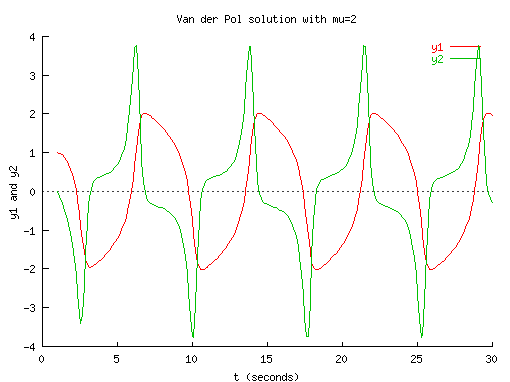Scientific Numerical Computing Ch is the simplest possible solution for numerical computing in the spirit of C. C was designed for Unix system programming. Scientific numerical computing was not the original design goal. For example, multi-dimensional arrays are difficult to handle in C. Ch extends C with salient numerical features in Fortran and special mathematical software packages such as MATLAB® and Mathematica® as shown in feature comparison of Ch with MATLAB/Mathematica and function comparison of Ch with MATLAB. Complicated problems in numerical analysis can often be solved with just one function call in Ch. Many features first implemented in Ch had been adopted in the latest ISO C standard called C99 ratified in 1999. The category of numerical analysis functions in Ch is listed below. Data Analysis and Statistics Data Interpolation and Curve Fitting Minimization or Maximization of Functions Polynomials Nonlinear Equations Ordinary Differential Equations Derivatives Integration Matrix Analysis Functions Matrix Decomposition Special Matrices Linear Equations Eigenvalues and Eigenvectors Fast Fourier Transforms Convolution and Filtering Cross Correlation Special Mathematical Functions You can find the demos from the link below. The following new features in Ch make it especially suitable for scientific numerical computing and rapid prototyping: Commonly used functions are built into Ch. For example, the following C/Ch program `````` #include #include #include int main() { float f=1; double d=2; complex z=3; double complex dz=3; f = sinf(f); d = sin(d); d = sinc(d); dz = sindc(dz); } ``` ``` can be written in Ch as `````` #include #include #include int main() { float f=1; double d=2; complex z=3; double complex dz=3; f = sin(f); d = sin(d); z = sin(z); dz = sin(dz); } ``` ``` where function sin() is one of many built-in generic functions. Complex is a built-in data type handled similar to that in Fortran. For example, `````` float f=90; complex z=complex(1,2); z = 2*f*z*sin(z)*atan(z); ``` ``` Support of IEEE 754 standard for binary floating-point arithmetic with metanumbers Inf, -Inf, NaN. Follows conventional mathematics in complex analysis, there is only one complex infinity and one complex-not-a-number. Complex numbers are defined in the entire complex domain with complex metanumbers ComplexInf and ComplexNaN. Different branches of multiple-valued complex functions can be obtained by mathematical functions with optional arguments. For example, the square root of -1 has two values of unit imaginary numbers i and -i. They can be obtained by generic function sqrt() in Ch as follows. ```> sqrt(-1.0) NaN > sqrt(complex(-1.0,0)) complex(0.0000,1.0000) > sqrt(complex(-1.0,0), 0) complex(0.0000,1.0000) > sqrt(complex(-1.0,0), 1) complex(-0.0000,-1.0000) > sqrt(-1, 0) complex(0.0000,1.0000) > sqrt(-1, 1) complex(-0.0000,-1.0000) > ``` Arrays of variable length. They include deferred-shape arrays, assumed-shape arrays, and pointer to assumed-shape arrays. The following example will clarify the concepts of these various array definitions. `````` void funct(int a[:][:], (*b)[:], n, m){ /* a: assumed-shape array */ /* b: pointer to array of assumed-shape */ int d; /* d: fixed-length array */ int e[n][m]; /* e: deferred-shape array */ int (*f)[:]; /* f: pointer to array of assumed-shape */ e = a; } int A, B; funct(A, B, 10, 20); funct(B, A, 85, 85); ``` ``` Arrays of adjustable range. The range of subscript for an index of array can be adjusted. For example, `````` int a[1:10], b[-5:5], c[0:10][1:10], d[1:10], e[n:m], f[n1:m1][1:m2]; extern int a[1:], b[-5:], c[0:][1:10]; int funct(int a[1:], int b[1:10], int c[1:], int d[1:10][0:20]); a = a+2; /* OK */ a = 90; /* Error: index out of range */ ``` ``` `````` void funct2(int a[0:]) { int i; int num=(int)shape(a); // num is 10, 10, 11 for a1, a2, a3, respectively for(i=0; i array double a = {1,2,3,4,5,6}, b > b = a*transpose(a) 14.0000 32.0000 32.0000 77.0000 > b*inverse(b) 1.0000 0.0000 0.0000 1.0000 > a = 100*a + 5 105.0000 205.0000 305.0000 405.0000 505.0000 605.0000 ``` In this example, the functions transpose()} and inverse() are used to calculate the transpose and inverse of a matrix, respectively. A function can return a computational array as well. For example, `````` array double fun(int i) { array double a; ... return a; } array double b; b = fun(3); ``` ``` The example below demonstrates the salient numerical features in Ch. `````` #include #include int main() { complex z; array double A, C = {1, 2, 3, 4, 5, 6, 7, 8, 9}; A = C*4 + sin(C); printf("A= \n%f \n", A); A = C * transpose(C)* inverse(C); printf("A= \n%f \n", A); z = complex(3,4); z = sin(2*z); printf("z = %f\n", z); } ``` ``` The output from the above code is as follows: `````` A= 4.841471 8.909297 12.141120 15.243198 19.041076 23.780585 28.656987 32.989358 36.412118 A= 64.000000 0.000000 32.000000 128.000000 0.000000 -64.000000 256.000000 0.000000 128.000000 z= complex(-416.462982,1431.113525) ``` ``` Pass by reference as in Fortran. The same syntax in C++ is used in Ch. For example, `````` void swap(int& i, int& j){ int tmp = i; i = j; j = tmp; } int main() { int i; int &j = i; int A, B; swap(A, B); /* pass by reference as in Fortran */ } ``` ``` A library of advanced numerical analysis functions. These advanced features for numerical analysis in Ch are very useful for applications in engineering and science. For example, a system of linear equations Ax = b can be solved using the code below. `````` array double A = {1,3,4, 39, 5, 4, 1, 3, 4}, b = {1,2,3}, x; linsolve(x, A, b); ``` ``` The user does not need to worry about the underlying optimization with fast and accurate numerical algorithms. Sample screen shots of applications using numerical functions in Ch are shown below. The source code for generating the graphical output and more Ch numerical computing demos are available. Using numerical computation features in Ch, interactive numerical analysis including Web-based integration and ordinary differential equation solving can be performed dynamically on-line.